Function Repository Resource:

# PolarDecomposition

Compute the polar decomposition of a matrix

Contributed by: Jan Mangaldan
 ResourceFunction["PolarDecomposition"][m] yields the polar decomposition for a numerical matrix m. The result is a list {q,s}, where q is a column orthonormal matrix and s is a Hermitian positive semidefinite matrix.

## Details

The original matrix m is equal to q.s.

## Examples

### Basic Examples (1)

Compute the polar decomposition for a 4×3 matrix:

 In:=Out=### Scope (2)

Compute the polar decomposition of a complex matrix:

 In:=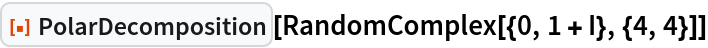Out=A matrix with entries having 20-digit precision:The polar decomposition is computed using the full 20-digit precision of the input:

 In:=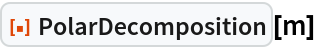Out=### Properties and Relations (2)

A random 5×4 matrix m: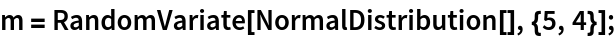Compute its polar decomposition:

 In:=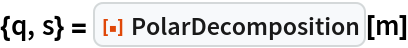Out=The columns of q are orthonormal:

 In:=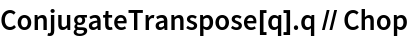Out=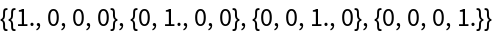The matrix s is Hermitian positive semidefinite:

 In:=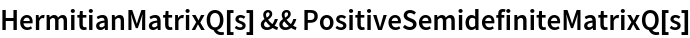Out=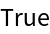The matrix s is equal to MatrixPower[ConjugateTranspose[m].m,1/2]:

 In:=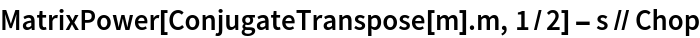Out=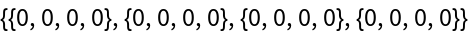m is equal to q.s:

 In:=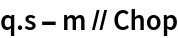Out=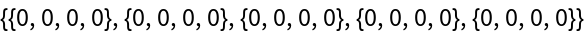If the matrix m is Hermitian positive semidefinite, then q is equal to the identity matrix, and s is equal to m:

 In:=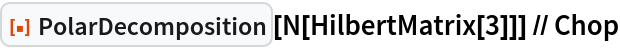Out=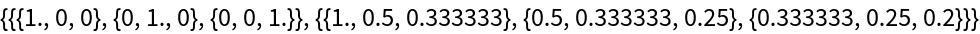In:=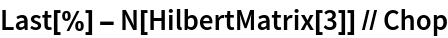Out=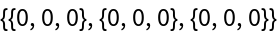### Possible Issues (1)

PolarDecomposition only works with approximate numerical matrices:

 In:=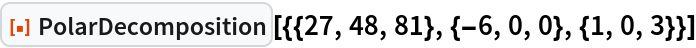Out=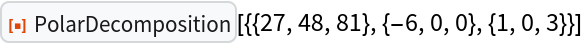In:=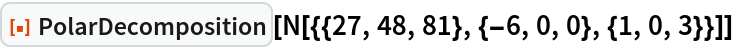Out=## Version History

• 1.0.0 – 17 May 2021